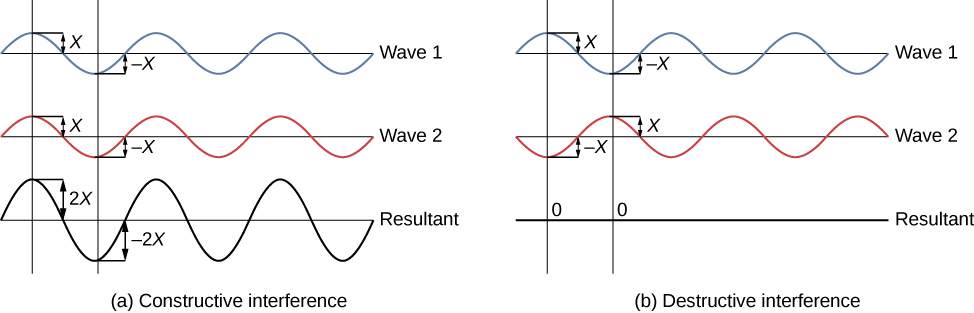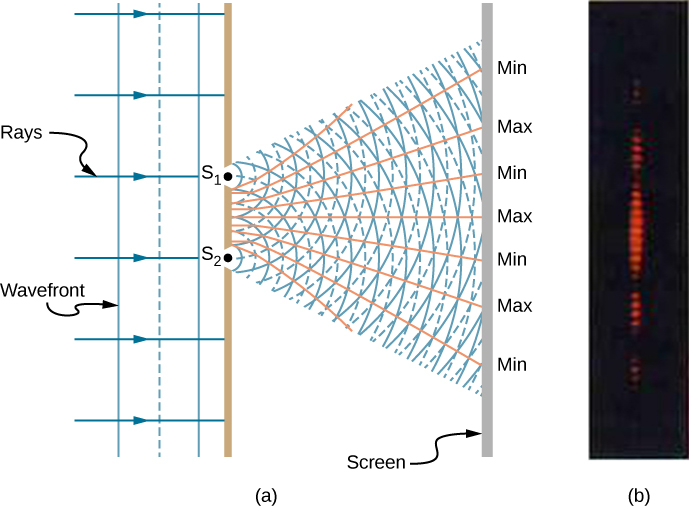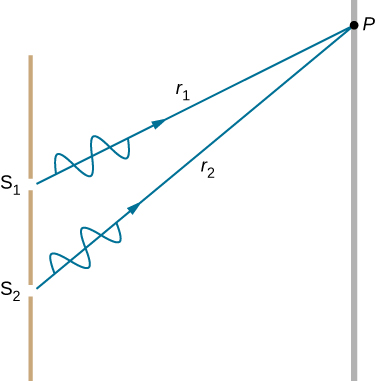# 3.1 Young's double-slit interference  (Page 2/5)

 Page 2 / 5

Young used sunlight, where each wavelength forms its own pattern, making the effect more difficult to see. In the following discussion, we illustrate the double-slit experiment with monochromatic    light (single $\lambda$ ) to clarify the effect. [link] shows the pure constructive and destructive interference of two waves having the same wavelength and amplitude.The amplitudes of waves add. (a) Pure constructive interference is obtained when identical waves are in phase. (b) Pure destructive interference occurs when identical waves are exactly out of phase, or shifted by half a wavelength.

When light passes through narrow slits, the slits act as sources of coherent waves and light spreads out as semicircular waves, as shown in [link] (a). Pure constructive interference occurs where the waves are crest to crest or trough to trough. Pure destructive interference occurs where they are crest to trough. The light must fall on a screen and be scattered into our eyes for us to see the pattern. An analogous pattern for water waves is shown in [link] . Note that regions of constructive and destructive interference move out from the slits at well-defined angles to the original beam. These angles depend on wavelength and the distance between the slits, as we shall see below.Double slits produce two coherent sources of waves that interfere. (a) Light spreads out (diffracts) from each slit, because the slits are narrow. These waves overlap and interfere constructively (bright lines) and destructively (dark regions). We can only see this if the light falls onto a screen and is scattered into our eyes. (b) When light that has passed through double slits falls on a screen, we see a pattern such as this.

To understand the double-slit interference pattern, consider how two waves travel from the slits to the screen ( [link] ). Each slit is a different distance from a given point on the screen. Thus, different numbers of wavelengths fit into each path. Waves start out from the slits in phase (crest to crest), but they may end up out of phase (crest to trough) at the screen if the paths differ in length by half a wavelength, interfering destructively. If the paths differ by a whole wavelength, then the waves arrive in phase (crest to crest) at the screen, interfering constructively. More generally, if the path length difference $\text{Δ}l$ between the two waves is any half-integral number of wavelengths [(1 / 2) $\lambda$ , (3 / 2) $\lambda$ , (5 / 2) $\lambda$ , etc.], then destructive interference occurs. Similarly, if the path length difference is any integral number of wavelengths ( $\lambda$ , 2 $\lambda$ , 3 $\lambda$ , etc.), then constructive interference occurs. These conditions can be expressed as equations:

$\text{Δ}l=m\lambda ,\phantom{\rule{0.8em}{0ex}}\text{for}\phantom{\rule{0.2em}{0ex}}m=0,\text{±}1,\text{±}2,\text{±}3\phantom{\rule{0.2em}{0ex}}\dots \phantom{\rule{0.2em}{0ex}}\text{(constructive interference)}$
$\text{Δ}l=\left(m+\frac{1}{2}\right)\lambda ,\phantom{\rule{0.8em}{0ex}}\text{for}\phantom{\rule{0.2em}{0ex}}m=0,\text{±}1,\text{±}2,\text{±}3\phantom{\rule{0.2em}{0ex}}\dots \phantom{\rule{0.2em}{0ex}}\text{(destructive interference)}$Waves follow different paths from the slits to a common point P on a screen. Destructive interference occurs where one path is a half wavelength longer than the other—the waves start in phase but arrive out of phase. Constructive interference occurs where one path is a whole wavelength longer than the other—the waves start out and arrive in phase.

## Summary

• Young’s double-slit experiment gave definitive proof of the wave character of light.
• An interference pattern is obtained by the superposition of light from two slits.

## Conceptual questions

Young’s double-slit experiment breaks a single light beam into two sources. Would the same pattern be obtained for two independent sources of light, such as the headlights of a distant car? Explain.

No. Two independent light sources do not have coherent phase.

Is it possible to create a experimental setup in which there is only destructive interference? Explain.

Why won’t two small sodium lamps, held close together, produce an interference pattern on a distant screen? What if the sodium lamps were replaced by two laser pointers held close together?

Because both the sodium lamps are not coherent pairs of light sources. Two lasers operating independently are also not coherent so no interference pattern results.

what is the difference between a molecule and atom
Atoms are single neutral particles. Molecules are neutral particles made of two or more atoms bonded together.
Manfred
what I'd dynamic propulsion
A body quadruples its momentum when its speed doubles.What was the initial speed in units of c.i.e..what was u/c ?
what is enthalpy?
a thermodynamic quantity equivalent to the total heat content of a system
RAMLA
proparty of tharmo dainamic
bloch
What is the meaning of Nuclear Fission?
what do you mean by dynamics single particles
عند قذف جسم إلى أعلى بسرعة إبتدائية فإنه سيصل إلى ارتفاع معين (أقصى ارتفاع) ثم يعود هابطاً نحو سطح الأرض .   إذا قُذِفَ جسم إلى أعلى ووجد أن سرعته 18 م / ث عندما قطع 1/4 المسافة التي تمثل أقصى ارتفاع سيصله فالمطلوب إيجاد السرعة التي قُذِف بها بالمتر / ث . إن هذه السرعة هي واحدة من الإجابات التالية
what is light
light is a kind of radiation That stimulates sight brightness a source of illumination.
kenneth
Electromagnet radiation creates space 7th, 8th, and 9th dimensions at the rate of c.
John
That is the reason that the speed of light is constant.
John
This creation of new space is "Dark Energy".
John
The first two sets of three dimensions, 1 through 6, are "Dark Matter".
John
As matter decays into luminous matter, a proton, a neutron, and an electron creat deuterium.
John
There are three sets of three protons, 9.
John
There are three sets of three neutrons, 9.
John
A free neutron decays into a proton, an electron, and a neutrino.
John
There are three sets of five neutrinoes, 15.
John
Neutrinoes are two dimensional.
John
A positron is composed of the first three dimensions.
John
An electron is composed of the second three dimensions.
John
What is photoelectric
light energy (photons) through semiconduction of N-P junction into electrical via excitation of silicon purified and cristalized into wafers with partially contaminated silicon to allow this N-P function to operate.
Michael
i.e. Solar pannel.
Michael
Photoelectric emission is the emission of electrons on a metal surface due to incident rays reflected on it
Benita
If you lie on a beach looking at the water with your head tipped slightly sideways, your polarized sunglasses do not work very well.Why not?
it has everything to do with the angle the UV sunlight strikes your sunglasses.
Jallal
this is known as optical physics. it describes how visible light, ultraviolet light and infrared light interact when they come into contact with physical matter. usually the photons or light upon interaction result in either reflection refraction diffraction or interference of the light.
Jallal
I hope I'm clear if I'm not please tell me to clarify further or rephrase
Jallal
what is bohrs model for hydrogen atom
hi
Tr
Hello
Youte
Hi
Nwangwu-ike
hi
Siddiquee
hi
Omar
helo
Mcjoi
what is the value of speed of light
1.79×10_¹⁹ km per hour
Swagatika
3×10^8
Benita
what r dwarf planet
what is energy
কাজের একক কী
Jasim
কাজের একক কী
Jasim
Energy is ability so capacity to do work.
kenneth
friction ka direction Kaise pata karte hai
friction is always in the opposite of the direction of moving object
Punia

#### Get Jobilize Job Search Mobile App in your pocket Now!By OpenStaxBy Nick SwainBy Katie MontroseBy OpenStaxBy Sarah WarrenBy Steve GibbsBy Madison ChristianBy Brooke DelaneyBy David GeltnerBy OpenStax